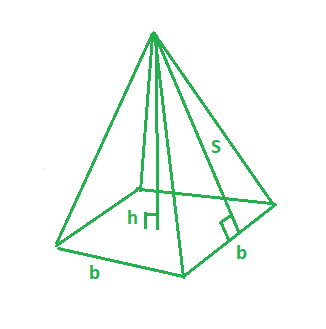Open in App
Not now

# Program to find the surface area of the square pyramid

• Last Updated : 07 Aug, 2022

Given the base length(b) and slant height(s) of the square pyramid. The task is to find the surface area of the Square Pyramid. A Pyramid with a square base, 4 triangular faces, and an apex is a square pyramid.In this figure,
b – base length of the square pyramid.
s – slant height of the square pyramid.
h – height of the square pyramid.
Examples:

Input: b = 3, s = 4
Output: 33

Input: b = 4, s = 5
Output: 56

Formula for calculating the surface are of the square pyramid with (b) base length and (s) slant height.Below is the implementation using the above formula:

## C++

 // CPP program to find the surface area // Of Square pyramid #include  using namespace std;   // function to find the surface area int surfaceArea(int b, int s) {       return 2 * b * s + pow(b, 2); }   // Driver program int main() {     int b = 3, s = 4;       // surface area of the square pyramid     cout << surfaceArea(b, s) << endl;     return 0; }

## Java

 // Java program to find the surface area // Of Square pyramid   import java.io.*;   class GFG {     // function to find the surface area static int surfaceArea(int b, int s) {       return 2 * b * s + (int)Math.pow(b, 2); }   // Driver program         public static void main (String[] args) {               int b = 3, s = 4;       // surface area of the square pyramid     System.out.println( surfaceArea(b, s));     } } //This code is contributed by anuj_67..

## Python 3

 # Python 3 program to find the  # surface area Of Square pyramid   # function to find the surface area def surfaceArea(b, s):       return 2 * b * s + pow(b, 2)   # Driver Code if __name__ == "__main__":     b = 3     s = 4       # surface area of the square pyramid     print(surfaceArea(b, s))   # This code is contributed  # by ChitraNayal

## C#

 // C# program to find the surface  // area Of Square pyramid using System;   class GFG  {   // function to find the surface area static int surfaceArea(int b, int s) {       return 2 * b * s + (int)Math.Pow(b, 2); }   // Driver Code public static void Main ()  {     int b = 3, s = 4;           // surface area of the square pyramid     Console.WriteLine(surfaceArea(b, s)); } }   // This code is contributed  // by inder_verma

## PHP

 

## Javascript

 

Output:

33

Time complexity: O(1)

Auxiliary Space: O(1)

My Personal Notes arrow_drop_up
Related Articles